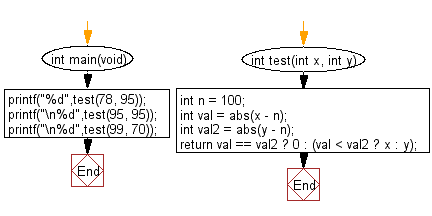﻿ C Program: Nearest integer to 100 from two integers# C Exercises: Check which number nearest to the value 100 among two given integers

## C-programming basic algorithm: Exercise-10 with Solution

Write a C program to check which number nearest to the value 100 among two given integers. Return 0 if the two numbers are equal.

C Code:

``````#include <stdio.h>
#include <stdlib.h>
int main(void){
printf("%d",test(78, 95));
printf("\n%d",test(95, 95));
printf("\n%d",test(99, 70));
}
int test(int x, int y)
{
int n = 100;
int val = abs(x - n);
int val2 = abs(y - n);
return val == val2 ? 0 : (val < val2 ? x : y);
}
``````

Sample Output:

```95
0
99
```

Pictorial Presentation:Flowchart:C Programming Code Editor:

What is the difficulty level of this exercise?

Test your Programming skills with w3resource's quiz.

﻿

## C Programming: Tips of the Day

Why doesn't a+++++b work?

printf("%d",a+++++b); is interpreted as (a++)++ + b according to the Maximal Munch Rule!.

++ (postfix) doesn't evaluate to an lvalue but it requires its operand to be an lvalue.

! 6.4/4 says the next preprocessing token is the longest sequence of characters that could constitute a preprocessing token"

Ref : https://bit.ly/3fdldUT